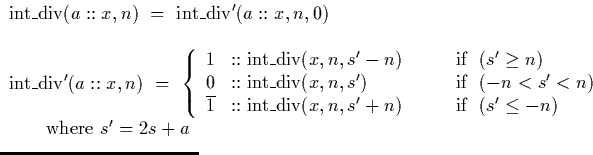Next: Signed Binary Average Up: Coding an algorithm in Previous: Coding an algorithm in

## Signed Binary Division by an Integer

In section 4.3.3, the algorithm for the division of a signed binary stream by an integer was defined as follows:The Haskell implementation of this algorithm is as follows:

-- sbIntDiv : Division of a signed binary stream by an integer
sbIntDiv :: SBinStream -> Int -> SBinStream
sbIntDiv _ 0    = undefined
sbIntDiv x 1    = x
sbIntDiv x (-1) = sbNegate x
sbIntDiv x n    = sbIntDiv' x n 0

-- sbIntDiv' : Auxiliary to sbIntDiv
sbIntDiv' :: SBinStream -> Int -> Int -> SBinStream
sbIntDiv' (a:x) n s = if (s' >= n)  then (  1:sbIntDiv' x n (s'-n)) else
if (s' <= -n) then ( -1:sbIntDiv' x n (s'+n))
else (  0:sbIntDiv' x n s')
where s' = 2*s+a


The algorithm and implementation are essentially the same. The only changes required are syntactic.

The lines starting with a double minus signed (--) are comments. The lines starting with a function name and followed by a double colon (::) are type definitions, and the remaining lines are the function definitions. The first three cases for sbIntDiv catch division by zero and avoid trivial divisions by one or minus one. The keyword where is a kind of postfix version of ML's let ... in ... statement. It allows us to define variables which are used in the main expression, and makes the code extremely readable.

The only remaining differences are that `cons' is represented using a single colon rather than the double one used in the algorithm description, and the three cases in the algorithm description are implemented using an if then statement.Next: Signed Binary Average Up: Coding an algorithm in Previous: Coding an algorithm in
Martin Escardo
5/11/2000